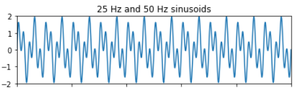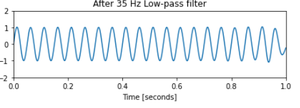# Noise Removal using Lowpass Digital Butterworth Filter in Scipy – Python

• Last Updated : 13 Jan, 2021

In this article, the task is to write a Python program for Noise Removal using Lowpass Digital Butterworth Filter.

## What is the noise?

Noise is basically the unwanted part of an electronic signal. It is often generated due to fault in design, loose connections, fault in switches etc.

## What to do if we have noise in our signal?

To remove unwanted signals/noise we use filters of different types and specifications. Generally in the industry we need to choose the best fit by testing it with the signal to pinpoint the best filter to be used for removing the noise in a given use case.

## What are we going to do now?

We are going to implement a Lowpass Digital Butterworth Filter now to remove the unwanted signal/noise of a combination of sinusoidal waves.

Filter Specifications:

• Signal made up of 25 Hz and 50 Hz
• Sampling frequency 1kHz.
• Order N=10 at 35Hz to remove 50Hz tone.

Step by Approach:

Step 1:Importing the libraries

## Python3

 `# import required library ` `import` `numpy as np ` `import` `scipy.signal as signal ` `import` `matplotlib.pyplot as plt `

Step 2:Defining the specifications

## Python3

 `# Specifications of the filter ` `f1 ``=` `25`  `# Frequency of 1st signal ` `f2 ``=` `50`  `# Frequency of 2nd signal ` `N ``=` `10`  `# Order of the filter ` ` `  ` `  `# Generate the time vector of 1 sec duration ` `t ``=` `np.linspace(``0``, ``1``, ``1000``)  ``# Generate 1000 samples in 1 sec ` ` `  `# Generate the signal containing f1 and f2 ` `sig ``=` `np.sin(``2``*``np.pi``*``f1``*``t) ``+` `np.sin(``2``*``np.pi``*``f2``*``t) `

Step 3:Plot the original signal with noise

## Python3

 `# Display the signal ` `fig, (ax1, ax2) ``=` `plt.subplots(``2``, ``1``, sharex``=``True``) ` `ax1.plot(t, sig) ` `ax1.set_title(``'25 Hz and 50 Hz sinusoids'``) ` `ax1.axis([``0``, ``1``, ``-``2``, ``2``]) ` ` `  `# Design the Butterworth filter using  ` `# signal.butter and output='sos' ` `sos ``=` `signal.butter(``50``, ``35``, ``'lp'``, fs``=``1000``, output``=``'sos'``) `

Output:Step 4:Plot of the signal after removing noise

## Python3

 `# Filter the signal by the filter using signal.sosfilt ` `# Use signal.sosfiltfilt to get output inphase with input ` `filtered ``=` `signal.sosfiltfilt(sos, sig) ` ` `  ` `  `# Display the output signal ` `ax2.plot(t, filtered) ` `ax2.set_title(``'After 35 Hz Low-pass filter'``) ` `ax2.axis([``0``, ``1``, ``-``2``, ``2``]) ` `ax2.set_xlabel(``'Time [seconds]'``) ` `plt.tight_layout() ` `plt.show() `

Output:Step 5: Implementation

## Python3

 `# import required library ` `import` `numpy as np ` `import` `scipy.signal as signal ` `import` `matplotlib.pyplot as plt ` ` `  `# Given ` `f1 ``=` `25`  `# Frequency of 1st signal ` `f2 ``=` `50`  `# Frequency of 2nd signal ` `N ``=` `10`  `# Order of the filter ` ` `  `# Generate the time vector of 1 sec duration ` `# START CODE HERE ### (≈ 1 line of code) ` `# Generate 1000 samples in 1 sec ` `t ``=` `np.linspace(``0``, ``1``, ``1000``) ` ` `  `# Generate the signal containing f1 and f2 ` `# START CODE HERE ### (≈ 1 line of code) ` `sig ``=` `np.sin(``2``*``np.pi``*``f1``*``t) ``+` `np.sin(``2``*``np.pi``*``f2``*``t) ` ` `  `# Display the signal ` `fig, (ax1, ax2) ``=` `plt.subplots(``2``, ``1``, sharex``=``True``) ` `ax1.plot(t, sig) ` `ax1.set_title(``'25 Hz and 50 Hz sinusoids'``) ` `ax1.axis([``0``, ``1``, ``-``2``, ``2``]) ` ` `  `# Design the Butterworth filter using signal.butter and output='sos' ` `# START CODE HERE ### (≈ 1 line of code) ` `sos ``=` `signal.butter(``50``, ``35``, ``'lp'``, fs``=``1000``, output``=``'sos'``) ` ` `  `# Filter the signal by the filter using signal.sosfilt ` `# START CODE HERE ### (≈ 1 line of code) ` `# Use signal.sosfiltfilt to get output inphase with input ` `filtered ``=` `signal.sosfiltfilt(sos, sig) ` ` `  ` `  `# Display the output signal ` `ax2.plot(t, filtered) ` `ax2.set_title(``'After 35 Hz Low-pass filter'``) ` `ax2.axis([``0``, ``1``, ``-``2``, ``2``]) ` `ax2.set_xlabel(``'Time [seconds]'``) ` `plt.tight_layout() ` `plt.show() `

Output:My Personal Notes arrow_drop_up
Recommended Articles
Page :